TaPAS  0.2
genepi.h File Reference

GENEPI Solver Interface. More...

#include <genepi/genepi-common.h>
#include <genepi/genepi-plugin-config.h>
#include <genepi/genepi-loader.h>

Go to the source code of this file.

## Macros

#define GENEPI_FLAG_SHOW_TIMERS   (0x01<<0)
Request the solver to evaluate the total execution time of function of the GENEPI API. More...

#define GENEPI_FLAG_SHOW_CACHE_INFO   (0x01<<1)
Request the solver to display information on the cache usage. More...

## Typedefs

typedef struct genepi_solver_st genepi_solver
Type of GENEPI solver. More...

typedef struct genepi_set_st genepi_set
Abstraction of Presburger definable set. More...

## Enumerations

enum  genepi_comparator
Comparison specifier. More...

enum  genepi_solver_type
Indicate the domain of variable used by a solver. More...

enum  genepi_info
Information string identifiers. More...

enum  genepi_io_error {
GENEPI_RW_ERR_IO = -1,
GENEPI_R_ERR_DATA_ERROR = -4
}
Error codes returned by the functions genepi_set_write and genepi_set_read. More...

## Functions

genepi_solvergenepi_solver_create (genepi_plugin *plugin, int flags, int cachesize, int number_of_cache_entries)
Creates a GENEPI solver. More...

void genepi_solver_delete (genepi_solver *solver)
Releases resources allocated by this solver. More...

genepi_solver_type genepi_solver_get_solver_type (genepi_solver *solver)
Gets the domain of variables used by the underlying plugin. More...

const char * genepi_solver_get_name (genepi_solver *solver)
Gets the name of the underlying plugin. More...

void genepi_solver_set_trace_file (genepi_solver *solver, FILE *output)
Enables/Disables GENEPI trace generator. More...

FILE * genepi_solver_get_trace_file (genepi_solver *solver)
Gets the current stream used for generate traces. More...

int genepi_solver_run_autotest (genepi_solver *solver)
Checks some tautologies with solver. More...

genepi_setgenepi_set_add_reference (genepi_solver *solver, genepi_set *X)
This function increments the number of references to the X set. More...

void genepi_set_del_reference (genepi_solver *solver, genepi_set *X)
Removes a reference to X. More...

genepi_setgenepi_set_top (genepi_solver *solver, int n)
Computes the complete relation of arity n. More...

genepi_setgenepi_set_top_N (genepi_solver *solver, int n)
Computes the restriction of the complete relation of arity n to positive or null integers. If the underlying plugin does not implement the top_N function then, depending on its solver type the function may raise an exception or not. More...

genepi_setgenepi_set_top_Z (genepi_solver *solver, int n)
Computes the restriction of the complete relation of arity n to integers. If the underlying plugin does not implement the top_Z function then, depending on its solver type the function may raise an exception or not. More...

genepi_setgenepi_set_top_P (genepi_solver *solver, int n)
Computes the restriction of the complete relation of arity n to positive real values. If the underlying plugin does not implement the top_P function then a generic function is used. More...

genepi_setgenepi_set_top_R (genepi_solver *solver, int n)
Computes the restriction of the complete relation of arity n to real values. More...

genepi_setgenepi_set_bot (genepi_solver *solver, int n)
Computes the empty relation of arity n. More...

genepi_setgenepi_set_linear_equality (genepi_solver *solver, const int *alpha, int alpha_size, int cst)
Computes the set of solutions of a linear equality. More...

genepi_setgenepi_set_linear_operation (genepi_solver *solver, const int *alpha, int alpha_size, genepi_comparator op, int c)
Computes the set of solutions of a linear operation. More...

genepi_setgenepi_set_union (genepi_solver *solver, genepi_set *X1, genepi_set *X2)
Computes the union of two relations. More...

genepi_setgenepi_set_intersection (genepi_solver *solver, genepi_set *X1, genepi_set *X2)
Computes the intersection of two relations. More...

genepi_setgenepi_set_complement (genepi_solver *solver, genepi_set *X)
Computes the complement of the set X. More...

genepi_setgenepi_set_project (genepi_solver *solver, genepi_set *X, const int *selection, int size)
Projects a relation on a subset of its columns. More...

genepi_setgenepi_set_invproject (genepi_solver *solver, genepi_set *X, const int *sel, int size)
Adds columns to the relation X. More...

genepi_setgenepi_set_apply (genepi_solver *solver, genepi_set *R, genepi_set *A)
Computes post (R, A) More...

genepi_setgenepi_set_applyinv (genepi_solver *solver, genepi_set *R, genepi_set *A)
Computes pre (R, A) More...

int genepi_set_compare (genepi_solver *solver, genepi_set *X1, genepi_comparator op, genepi_set *X2)
Compares X1 and X2 according to op. More...

int genepi_set_equal (genepi_solver *solver, genepi_set *X1, genepi_set *X2)
Checks the equality (mutual inclusion) of X1 and X2. More...

int genepi_set_is_empty (genepi_solver *solver, genepi_set *X)
Checks if X is empty. More...

int genepi_set_is_full (genepi_solver *solver, genepi_set *X)
Checks if X is the complete relation. More...

int genepi_set_is_finite (genepi_solver *solver, genepi_set *X)
Checks if the set is finite. More...

int genepi_set_depend_on (genepi_solver *solver, genepi_set *X, const int *sel, int selsize)
Checks if the relation X depends on a subset of its column. More...

void genepi_set_get_solutions (genepi_solver *solver, genepi_set *X, int ***psolutions, int *psize, int max)
Computes an explicit representation of X. More...

void genepi_set_display_all_solutions (genepi_solver *solver, genepi_set *X, FILE *output, const char *const *varnames)
Displays all solutions of the set X. More...

void genepi_set_display_data_structure (genepi_solver *solver, genepi_set *X, FILE *output)
Displays internal representation of X. More...

int genepi_set_get_width (genepi_solver *solver, genepi_set *X)
Arity of the relation represented by X. More...

int genepi_set_get_data_structure_size (genepi_solver *solver, genepi_set *X)
Size of the data structure encoding X. More...

int genepi_set_is_solution (genepi_solver *solver, genepi_set *X, const int *x, int x_size, int xden)
Checks if x/xden belongs to X. More...

int genepi_set_get_one_solution (genepi_solver *solver, genepi_set *X, int *x, int x_size)
Gets one element in X. More...

int genepi_set_write (genepi_solver *solver, FILE *output, genepi_set *set)
Dump the set X onto the output stream. More...

int genepi_set_read (genepi_solver *solver, FILE *input, genepi_set **pset)
Load on the input stream a serialized set. More...

genepi_setgenepi_set_transform (genepi_solver *solver, genepi_set *X, int *indices, int nb_indices, int *cst, int nb_cst)
Transformation of the relation X. More...

int genepi_set_preorder (genepi_solver *solver, genepi_set *X1, genepi_set *X2)
Evaluate the pre-order over sets. More...

## Detailed Description

GENEPI Solver Interface.

This module is the part of GENEPI used to build Presburger definable sets. Sets are built via a solver which relates the client code of GENEPI to a dynamically loaded plugin.

# Solvers

Once a plugin has been selected and loaded with the GENEPI loader a solver can be created using the create function. GENEPI solvers can use a cache for some Presburger operations (each solver has its proper cache). The size of the cache is specified (in terms of the number of bytes or entries of the table) at creation time.

At any time the client code can indicate the solver to produce a trace of GENEPI calls (cf. set_trace_file). The trace is generated in a FILE which can be reinterpreted by the DIJO tool. The description of the file generated by the solver is given in genepi/genepi-trace.h.

The client code can request GENEPI to verify the plugin by checking some tautologies (cf. autotest). This function can be useful when integrating a new plugin.

# Sets

A solver is then used to build sets of solutions of Presburger formulas. These sets are n-ary where n is the number of variables appearing in the formula. GENEPI sets are only relation which means that no link exists between the column of relations and the variable of a formula. Indeed the notion of formula does not appear in GENEPI. The API furnishes a minimal kernel of functions permitting the computation the solutions of a Presburger formula.

Since variable are not attached to columns of relation the client code has to take care of formulas built over different sets of variables. For example, if one wants to compute the solutions of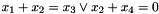one can have two approaches:

• compute the solutions of each equation as relation of arity 4 and then compute the union.
• compute the solutions of the first equation as a ternary relation and the second as a binary relation. Then enlarge both relations to obtain two relations of arity 4 and make the union. In this solution, when a relation is enlarged, one has to take care to putin the same column for both relations.

The GENEPI interface offers the following functionalities (each one depends on the abilities of the underlying plugin):

Elementary relations are built with the following functions:
•if built using bot function
•whereis a domain for variables with the top, top_N, top_Z, top_P and top_R.
•where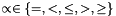are built using linear_operation. Equality can be built directly using linear_equality.
Boolean operations:
Basic operations on set are done with the functions: complement, union and intersection.
Column manipulations use two functions:
• project which projects a relation on a subset of its column. This function is used to implement existential quantification.
• invproject which add columns to a relation.
pre and post computations:
Basically this library has been designed for the FASTer model-checker. Two functions permit to compute for a given transition relation the successors or the predecessors of a given set; set apply and applyinv.
Some elementary properties can be checked on relation:
• inclusion and equality of two relations can be checked with, resp., compare and equal functions.
• emptiness, completeness and finiteness can be checked with the functions, respectively, is_empty, is_full and is_finite.
• dependency to one or several columns is checked by depend_on.
Explicit solutions:
It is possible to get one or more elements belonging to a computed set.
• get_one_solution returns one element if the set is not empty.
• is_solution checks if a vector belongs to a set.
• get_solutions is used to retrieve all solutions in case of finite sets or a subset of solutions in case of infinite sets.
Serialization:
One can serialize any compute set into a standard C FILE-stream. Use write to save a set and use read to reload it. Of course these functions must not be used with the DIJO traces.

Definition in file genepi.h.

## Macro Definition Documentation

 #define GENEPI_FLAG_SHOW_CACHE_INFO   (0x01<<1)

Request the solver to display information on the cache usage.

Before the deletion of the solver (genepi_solver_delete), if its cache is enabled then some statistics related to the operation cache usage is displayed on the standard output.

genepi_solver_create

Definition at line 218 of file genepi.h.

 #define GENEPI_FLAG_SHOW_TIMERS   (0x01<<0)

Request the solver to evaluate the total execution time of function of the GENEPI API.

If this flag is specified when a solver is created then each time a genepi_set_X function is called (only function requiring important computation time i.e. projection, union, ...), GENEPI maintains a counter of number of calls and the total time used to execute the function. The execution time is evaluated using the standard clock (3) function.

When the solver is deleted all this informations are gathered and displayed on standard output.

genepi_solver_create

Definition at line 207 of file genepi.h.

## Typedef Documentation

 typedef struct genepi_set_st genepi_set

Abstraction of Presburger definable set.

Variables of this type are relations definable by a Presburger formula. This type is manipulated using a GENEPI solver and the data structure used to encode sets depend on the plugin used by the solver.

Definition at line 190 of file genepi.h.

 typedef struct genepi_solver_st genepi_solver

Type of GENEPI solver.

A GENEPI solver is used to encode Presburger definable sets by means of genepi_sets. The data structure used to encode such sets depend on a plugin specified at the creation time of the solver.

Definition at line 180 of file genepi.h.

## Enumeration Type Documentation

 enum genepi_comparator

Comparison specifier.

This enumeration is used to indicate to certain function a comparison relation. EQ means "equal", LT means "less than", GT means "greater than", LEQ means "less than" and GEQ means "greater than". Of course, the interpretation depends on the underlying set.

genepi_set_compare and genepi_set_linear_operation

Definition at line 230 of file genepi.h.

 enum genepi_info

Information string identifiers.

This enumeration is used to retrieve informations about the underlying plugin used by a solver.

genepi_solver_get_info

Definition at line 263 of file genepi.h.

 enum genepi_io_error

Error codes returned by the functions genepi_set_write and genepi_set_read.

Enumerator
GENEPI_RW_ERR_IO

An has occurred on the stream at the system level.

The client code tries to load a set saved with a plugin different of the one currently used.

The client code tries to load a set saved with another version of the plugin currently used.

GENEPI_R_ERR_DATA_ERROR

Malformed stream: data don't respect the serialization format expected by GENEPI or by the underlying plugin.

Definition at line 275 of file genepi.h.

 enum genepi_solver_type

Indicate the domain of variable used by a solver.

GENEPI_N_SOLVER indicates that variables are positive or null integers GENEPI_Z_SOLVER indicates that variables are are positive, negative or null integer GENEPI_P_SOLVER indicates that variables are positive or null real numbers GENEPI_R_SOLVER indicates that variables are rational numbers

Definition at line 248 of file genepi.h.

## Function Documentation

 genepi_set* genepi_set_add_reference ( genepi_solver * solver, genepi_set * X )

This function increments the number of references to the X set.

Parameters
 solver the GENEPI solver X the considered set
Precondition
solver != NULL
X != NULL
number of references to X > 0
Returns
X
 genepi_set* genepi_set_apply ( genepi_solver * solver, genepi_set * R, genepi_set * A )

Computes post (R, A)

This function applies the relation R to the set A. If n is the width of A, the function assumes that 2*n is the width of R. The function computes the set of vectors defined by:Note the interleaving of primed and not primed variables and also note that the primed variable precedes its non-primed version.

Parameters
 solver the GENEPI solver R the transition relation A the relation on which R is applied.
Precondition
genepi_set_get_width (solver, R) = 2 * genepi_set_get_width (solver, A)
Returns
a pointer to the structure encoding post(R,A)
 genepi_set* genepi_set_applyinv ( genepi_solver * solver, genepi_set * R, genepi_set * A )

Computes pre (R, A)

This function is similar to apply but instead of computing the image of A by the relation R, it computes the set whose image is A by R. Formally, the function computes the set:Note the interleaving of primed and not primed variables and also note that the primed variable precedes its non-primed version.

Parameters
 solver the GENEPI solver R the transition relation; A the image by R of the computed relation.
Precondition
genepi_set_get_width (solver, R) = 2 * genepi_set_get_width (solver, A)
Returns
a pointer to the structure encoding pre(R,A)
 genepi_set* genepi_set_bot ( genepi_solver * solver, int n )

Computes the empty relation of arity n.

Parameters
 solver the GENEPI solver n the arity of the computed relation
Precondition
the plugin implements the bot function
n > 0
Returns
the encoding ofint genepi_set_compare ( genepi_solver * solver, genepi_set * X1, genepi_comparator op, genepi_set * X2 )

Compares X1 and X2 according to op.

The function returns true if X1 and X2 verify the relation op where the genepi_comparator op is interpreted in the context of sets inclusion.

Parameters
 solver the GENEPI solver X1 first operand of the comparison X2 second operand of the comparison op comparison specifier
Precondition
solver != NULL
the plugin implements the compare function
genepi_set_get_width (solver, X1) == genepi_set_get_width (solver, X2)
Returns
a non null value if X1 op X2
 genepi_set* genepi_set_complement ( genepi_solver * solver, genepi_set * X )

Computes the complement of the set X.

This function computes the completement of X in the domain of variables i.e. either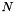or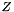.

Parameters
 solver the GENEPI solver X the set to complement.
Precondition
solver != NULL
the plugin implements the complement function
X != NULL
Returns
the encoding of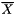void genepi_set_del_reference ( genepi_solver * solver, genepi_set * X )

Removes a reference to X.

This function decrements the number of references to the X set. If this is equal to 0 then all memory related to X is freed.

Parameters
 solver the GENEPI solver X the considered set
Precondition
solver != NULL
X != NULL
number of references to X > 0
 int genepi_set_depend_on ( genepi_solver * solver, genepi_set * X, const int * sel, int selsize )

Checks if the relation X depends on a subset of its column.

X is said to be dependent of columns(whereis such that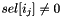) if the projection of X these columns is not the complete relation of arity n.

Parameters
 solver the GENEPI solver X the relation on which we check dependency. sel an array of Booleans identifying the columns on which the dependency is checked. selsize the number of cells in sel.
Precondition
solver != NULL
sel != NULL
selsize == genepi_set_get_width (solver, X)
 void genepi_set_display_all_solutions ( genepi_solver * solver, genepi_set * X, FILE * output, const char *const * varnames )

Displays all solutions of the set X.

If the underlying plugin implements the function display_all_solutions then this function displays all elements of X. If X is infinite and the plugin does not display a symbolic representation of X then the program can enter an infinite loop.

Parameters
 solver the GENEPI solver X the considered relation output the stream on which the solutions are displayed. varnames the names labelling the column of the relation; the size of this array must be equal to the arity of the relation.
Precondition
solver != NULL
X != NULL
output != NULL
varnames != NULL
 void genepi_set_display_data_structure ( genepi_solver * solver, genepi_set * X, FILE * output )

Displays internal representation of X.

If the underlying plugin implements the display_data_structure then this function displays the data structure used to encode X.

Parameters
 solver the GENEPI solver X the considered relation output the stream on which the data structure is displayed
Precondition
solver != NULL
X != NULL
output != NULL
 int genepi_set_equal ( genepi_solver * solver, genepi_set * X1, genepi_set * X2 )

Checks the equality (mutual inclusion) of X1 and X2.

Parameters
 solver the GENEPI solver X1 first argument of the comparison X2 second argument of the comparison
Returns
a non null value is the two sets are equal.
Remarks
is strictly equivalent to : genepi_set_compare (X1, GENEPI_EQ, X2)
genepi_set_compare
 int genepi_set_get_data_structure_size ( genepi_solver * solver, genepi_set * X )

Size of the data structure encoding X.

The result of this function is an integer indicating a size of the data structure required to encode X. This is not necessary a number of bytes (which have no sense with shared structures) but if the size of X is greater than the size of Y then X requires much memory resources than Y.

Parameters
 solver the GENEPI solver X the considered relation.
Precondition
solver != NULL
the plugin implements the get_data_structure_size function
X != NULL
Returns
an integer representing a size of the underlying encoding of X.
 int genepi_set_get_one_solution ( genepi_solver * solver, genepi_set * X, int * x, int x_size )

Gets one element in X.

The function fills the given vector x with an element of X if it is not empty. The way the function selects x is not specified and depends on the plugin implementation.

Parameters
 solver the GENEPI solver X the considered set x the vector which receives the element of X x_size the size of x
Precondition
solver != NULL
the plugin implements the get_one_solution function.
x_size == genepi_set_get_width (solver, X)
Postcondition
result >= 0
Return values
 0 if X is empty. the common denominator of the solution x
 void genepi_set_get_solutions ( genepi_solver * solver, genepi_set * X, int *** psolutions, int * psize, int max )

Computes an explicit representation of X.

If the plugin implements the function get_solutions then this function computes an array of vectors representing elements of the set X. Each vector is an array of integers allocated by the function. If X is an infinite set then max parameter is used to indicate the maximal number of vectors to return. The way the function choose the vectors is not specifed.

The vectors are returned via the psolutions parameter and the number of vectors is set into the variable pointed by psize.

If the max if negative then all the solutions are returned.

Parameters
 solver the GENEPI solver X the set X psolutions the address where the vectors are stored psize the address of the integer receiving the number of solution max the maximal number of solutions.
Precondition
solver != NULL
X != NULL
psolutions != NULL
psize != NULL
max >= 0 || genepi_set_is_finite (solver, X))
 int genepi_set_get_width ( genepi_solver * solver, genepi_set * X )

Arity of the relation represented by X.

Parameters
 solver the GENEPI solver X the considered relation.
Precondition
solver != NULL
the plugin implements the get_width function
X != NULL
Returns
the number of columns of the relation X.
 genepi_set* genepi_set_intersection ( genepi_solver * solver, genepi_set * X1, genepi_set * X2 )

Computes the intersection of two relations.

This function has to compute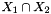where relations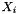are assumed to be of same arity.

Parameters
 solver the GENEPI solver X1 the first operand of the intersection X2 the second operand of the intersection
Precondition
solver != NULL
the plugin implements the intersection function
genepi_set_get_width (solver, X1) == genepi_set_get_width (solver, X2)
Returns
the encoding ofgenepi_set* genepi_set_invproject ( genepi_solver * solver, genepi_set * X, const int * sel, int size )

Adds columns to the relation X.

This function add columns to X according to the array of Booleans selection. The arity of the resulting relation is size and the relation itself is defined by: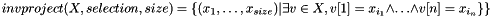where n is the arity of X,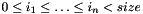and for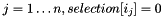.

Parameters
 solver the GENEPI solver X the relation where new columns are added sel the specification of new columns size number of column in the result.
Precondition
the plugin implements the invproject function
selection != NULL
size >= genepi_set_get_width (solver, X)
Returns
the encoding of X extended with additionnal columns specified in selection.
 int genepi_set_is_empty ( genepi_solver * solver, genepi_set * X )

Checks if X is empty.

This function checks if the set represented by X is the empty relation of arity get_width (X).

Parameters
 solver the GENEPI solver X the considered set
Precondition
solver != NULL
the plugin implements the genepi_plugin_is_empty is_empty function
X != NULL
Returns
a non null value if X is empty.
 int genepi_set_is_finite ( genepi_solver * solver, genepi_set * X )

Checks if the set is finite.

Parameters
 solver the GENEPI solver X the considered set
Precondition
solver != NULL
the plugin implements the is_finite function
X != NULL
Returns
a non null value is the set X is finite
 int genepi_set_is_full ( genepi_solver * solver, genepi_set * X )

Checks if X is the complete relation.

This function checks if the set represented by X is the complete relation of arity get_width (X).

Parameters
 solver the GENEPI solver X the tested relation
Precondition
solver != NULL
the plugin implements the is_full function
X != NULL
Returns
a non null value if X is complete
 int genepi_set_is_solution ( genepi_solver * solver, genepi_set * X, const int * x, int x_size, int xden )

Checks if x/xden belongs to X.

Parameters
 solver the GENEPI solver X the considered relation x the vector whose belonging is tested. x_size the size of x xden a common denominator of components of x
Precondition
solver != NULL
x != NULL
x_size == genepi_set_get_width (solver, X)
xden > 0
Returns
a non null value if x /xden\ is an element of X.
 genepi_set* genepi_set_linear_equality ( genepi_solver * solver, const int * alpha, int alpha_size, int cst )

Computes the set of solutions of a linear equality.

This function is a shortcut for: genepi_set_linear_operation (solver, alpha, alpha_size, GENEPI_EQ, cst)

Parameters
 solver the GENEPI solver alpha the array of coefficient of the linear operation alpha_size the number of coefficients cst the constant term of the operation
Returns
the solutions of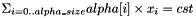genepi_set* genepi_set_linear_operation ( genepi_solver * solver, const int * alpha, int alpha_size, genepi_comparator op, int c )

Computes the set of solutions of a linear operation.

According to the value of op this function returns the set of solutions of the linear operation: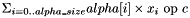with op interpreted on natural numbers.

Parameters
 solver the GENEPI solver alpha the array of coefficient of the linear operation alpha_size the number of coefficients c the constant term of the operation op the identifier of the operation
Precondition
solver != NULL
the plugin implements the linear_operation function
alpha != NULL && alpha_size > 0
Returns
the solutions of the specified linear operation.
 int genepi_set_preorder ( genepi_solver * solver, genepi_set * X1, genepi_set * X2 )

Evaluate the pre-order over sets.

This function implements the pre-order relationsuch that for all sets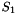,and:

•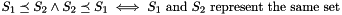••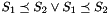Parameters
 X1 first operand X2 second operand
Precondition
X1 != NULL
X2 != NULL
Returns
a non null value of X1X2
 genepi_set* genepi_set_project ( genepi_solver * solver, genepi_set * X, const int * selection, int size )

Projects a relation on a subset of its columns.

This function computes the projection of X on the columns selected by the array of Booleans selection. The function computes:whereand for.

Parameters
 solver the GENEPI solver X the relation to project selection the selection of columns of X which must be kept by the projection. size the number of Booleans in selection.
Precondition
the plugin implements the project function
selection != NULL
size == genepi_set_get_width (solver, X)
Returns
the encoding of the projection of X according to the selected columns in selection.
 int genepi_set_read ( genepi_solver * solver, FILE * input, genepi_set ** pset )

Load on the input stream a serialized set.

This function is used to reload a genepi_set serialized with the genepi_set_write write function. The loaded set is putted in *pset. The function returns the number of bytes actually readed. If a negative or null value is returned an error has occurred and the returned value has to be interpreted as genepi_io_error.

Parameters
 solver the current GENEPI solver input the stream where the set is loaded pset a pointer to the variable receiving the loaded set.
Precondition
solver != NULL
the underlying plugin must implement the read_set function.
input != NULL
pset != NULL
Returns
the number of bytes read on input or an error code
genepi_set_write
 genepi_set* genepi_set_top ( genepi_solver * solver, int n )

Computes the complete relation of arity n.

Parameters
 solver the GENEPI solver n the arity of the computed relation
Precondition
solver != NULL
the plugin implements the top function
n > 0
Returns,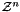,or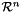depending on the result of get_solver_type.
 genepi_set* genepi_set_top_N ( genepi_solver * solver, int n )

Computes the restriction of the complete relation of arity n to positive or null integers. If the underlying plugin does not implement the top_N function then, depending on its solver type the function may raise an exception or not.

Parameters
 solver the GENEPI solver n the arity of the computed relation
Precondition
solver != NULL
n > 0
Returns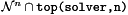genepi_set* genepi_set_top_P ( genepi_solver * solver, int n )

Computes the restriction of the complete relation of arity n to positive real values. If the underlying plugin does not implement the top_P function then a generic function is used.

Parameters
 solver the GENEPI solver n the arity of the computed relation
Precondition
solver != NULL
n > 0
Returns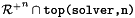genepi_set* genepi_set_top_R ( genepi_solver * solver, int n )

Computes the restriction of the complete relation of arity n to real values.

Parameters
 solver the GENEPI solver n the arity of the computed relation
Precondition
solver != NULL
n > 0
Returns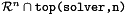genepi_set* genepi_set_top_Z ( genepi_solver * solver, int n )

Computes the restriction of the complete relation of arity n to integers. If the underlying plugin does not implement the top_Z function then, depending on its solver type the function may raise an exception or not.

Parameters
 solver the GENEPI solver n the arity of the computed relation
Precondition
solver != NULL
n > 0
Returns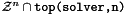genepi_set* genepi_set_transform ( genepi_solver * solver, genepi_set * X, int * indices, int nb_indices, int * cst, int nb_cst )

Transformation of the relation X.

This function produces a new relation Y from X in the following way:

• the arity of Y is nb_indices
• for all i in { 0, ..., nb_indices}: . if indices[i] >= genepi_set_get_width (X) then Y[i] is set to \ genepi_set_top top() . if 0 <= indices[i] < genepi_set_get_width (X) then Y[i] = X[indices[i]]. . if indices[i] < 0 then Y[i] is to the constant cst[abs(indices[i])-1] given by the array cst.
Parameters
 solver the current GENEPI solver X the relation to transform indices specification of new columns nb_indices width of the result cst constants use in the specification of new columns nb_cst number of constant used
Precondition
solver!= NULL
X != NULL
for all i, indices[i] < 0 => -indices[i]-1 < nb_cst
 genepi_set* genepi_set_union ( genepi_solver * solver, genepi_set * X1, genepi_set * X2 )

Computes the union of two relations.

This function has to compute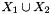where relationsare assumed to be of same arity.

Parameters
 solver the GENEPI solver X1 the first operand of the union X2 the second operand of the union
Precondition
solver != NULL
the plugin implements the union function
genepi_set_get_width (solver, X1) == genepi_set_get_width (solver, X2)
Returns
the encoding ofint genepi_set_write ( genepi_solver * solver, FILE * output, genepi_set * set )

Dump the set X onto the output stream.

This function can be used to serialize a set X onto an output stream. The set X can be NULL; in this case the NULL value is written on the stream. The function returns the number of bytes actually written. If the result is negative or null then an error has occured and the returned value has to be interpreted as a genepi_io_error.

Parameters
 solver the current GENEPI solver output the stream where data are written. set the dumped GENEPI set
Precondition
solver != NULL
the underlying plugin must implement the write_set function.
output != NULL
Returns
the number of bytes written on output or an error code
 genepi_solver* genepi_solver_create ( genepi_plugin * plugin, int flags, int cachesize, int number_of_cache_entries )

Creates a GENEPI solver.

This function creates a solver based on the plugin (cf. genepi/genepi-plugin.h) used to encode the Presburger definable sets.

The size of the operation cache can be specified in two ways either by the total size in bytes of the table, either by the number of its entries. The number of entries is converted into a number of bytes and then the biggest size is selected. If the cache size is 0 or if the underlying plugin does not implement one of the function cache_hash or cache_equal then the cache is disabled.

flags are used to customize the behaviors of the solver and are formed by a bitwise disjunction of macros GENEPI_FLAG_X .

Parameters
 plugin the plugin encoding the Presburger sets flags customization flags cachesize the size in bytes of the operation cache number_of_cache_entries the size in number of entries of the operation cache
Returns
a pointer to a new solver using plugin as set encoding.
 void genepi_solver_delete ( genepi_solver * solver )

Releases resources allocated by this solver.

The underlying plugin is not deleted (this operation depends on the loader). If the flags specified when the solver has been created contain SHOW_TIMERS then the execution times for each function of the API is displayed. If flags contain SHOW_CACHE_INFO then information related to the cache on GENEPI operations is displayed.

Parameters
 solver the GENEPI solver
Precondition
solver != NULL
 const char* genepi_solver_get_name ( genepi_solver * solver )

Gets the name of the underlying plugin.

Parameters
 solver the GENEPI solver

This function returns the name of the underlying GENEPI plugin.

Precondition
solver != NULL
Returns
a pointer to the path to plugin binary file
 genepi_solver_type genepi_solver_get_solver_type ( genepi_solver * solver )

Gets the domain of variables used by the underlying plugin.

This function returns the domain of variables used by the solver. If the plugin doesn't supply this information we assume that this domain is the set of non-negative integers(i.e. GENEPI_N_SOLVER is returned).

Parameters
 solver the GENEPI solver
Precondition
solver != NULL
Returns
the domain of variables of solver.
 FILE* genepi_solver_get_trace_file ( genepi_solver * solver )

Gets the current stream used for generate traces.

Parameters
 solver the GENEPI solver

This function returns the FILE pointer currently used as output for the GENEPI trace generator. If the function returns NULL it means that no trace is generated.

Precondition
solver != NULL
Returns
the pointer to the trace stream or NULL if the generator is disabled.
 int genepi_solver_run_autotest ( genepi_solver * solver )

Checks some tautologies with solver.

Parameters
 solver the GENEPI solver

This function runs tests on the 'solver'. Two kind of tests are used. If the plugin provides its own test function, then this one is called. Then, generic tests are executed using the GENEPI functions.

Precondition
solver != NULL
Returns
a non-null value is all tests are passed and 0 if one test fail.
 void genepi_solver_set_trace_file ( genepi_solver * solver, FILE * output )

Enables/Disables GENEPI trace generator.

This procedure sets or changes the FILE where GENEPI traces are generated. If a file is already in use then this stream is 'fflushe'd (but not 'fclose'd) and associated data structures are cleaned. The 'output' might be NULL, in which case no more traces are generated.

Parameters
 solver the GENEPI solver output the stream on whoich traces are generated
Precondition
solver != NULL